# How does Successive Approximation (SAR) ADC Work and Where is it best used?

Published  October 30, 2020   0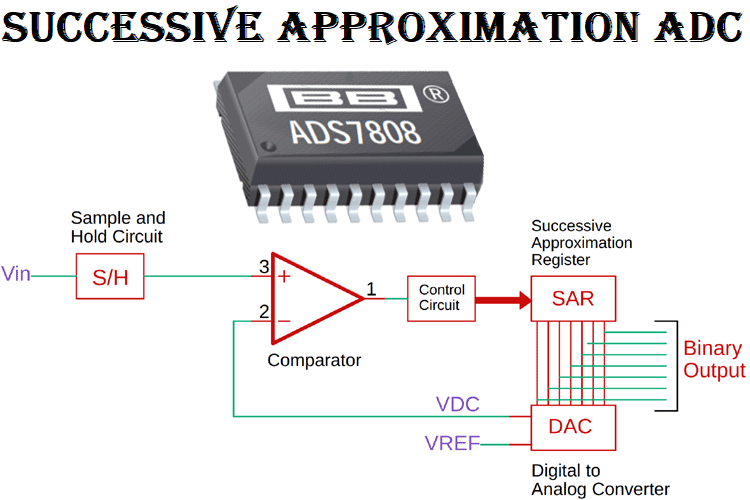### What is a Successive Approximation ADC?

The Successive Approximation ADC is the ADC of choice for low-cost medium to high-resolution applications, the resolution for SAR ADCs ranges from 8 - 18 bits, with sample speeds up to 5 mega-samples per second (Msps). Also, it can be constructed in a small form factor with low power consumption, which is why this type of ADC is used for portable battery-powered instruments.

As the name implies, this ADC applies a binary search algorithm to convert the values, which is why the internal circuitry may be running at several MHZ but the actual sample rate is much less due to the Successive Approximation algorithm. We discuss more about it later in this article.

### Working of Successive Approximation ADC

The cover image shows the basic successive approximation ADC circuit. But to understand the working principle a little better, we are going to use a 4-bit version of it. The image below shows exactly that.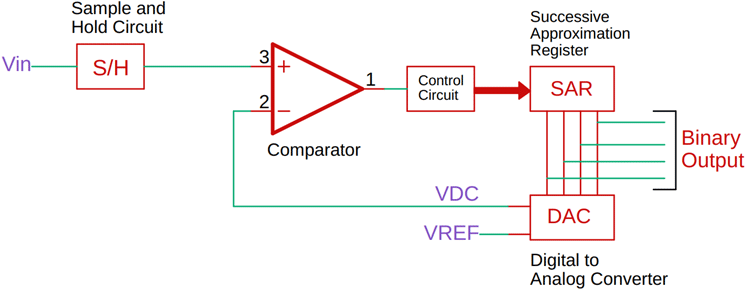As you can see, this ADC consists of a comparator, a digital to analog converter, and a successive approximation register along with the control circuit. Now, whenever a new conversation starts, the sample and hold circuit samples the input signal. And that signal is compared with the specific output signal of the DAC.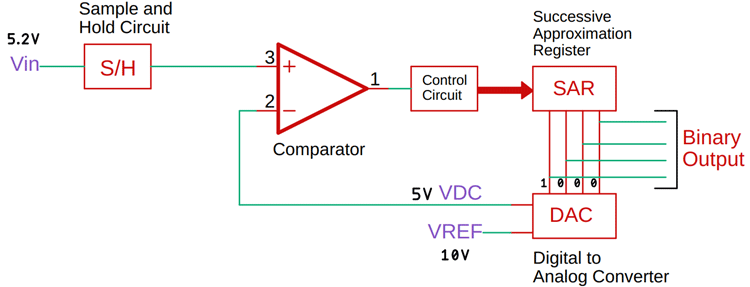Now let's say, the sampled input signal is 5.8V. The reference of the ADC is 10V. When the conversion starts, the successive approximation register sets the most significant bit to 1 and all other bits to zero. This means the value becomes 1, 0, 0, 0, which means, for a 10V reference voltage, the DAC will produce a value of 5V which is half of the reference voltage. Now this voltage will be compared to the input voltage and based on the comparator output, the output of the successive approximation register will be changed. The image below will clarify it more. Further, you can look at a generic reference table for more details on DAC. Previously we have made many projects on ADCs and DACs, you can check those out for more information.

This means if Vin is greater than the output of the DAC, the most significant bit will stay as it is, and the next bit will be set for a new comparison. Otherwise, if the input voltage is less than the DAC value, the most significant bit will be set to zero, and the next bit will be set to 1 for a new comparison. Now if you see the below image, the DAC voltage is 5V and as it is less than the input voltage, the next bit before the most significant bit will set to one, and other bits will set to zero, this process will continue until the value closest to the input voltage reaches.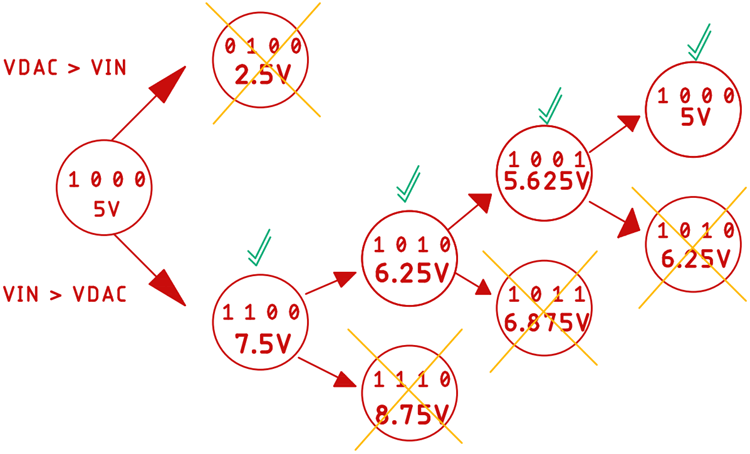This is how the successive approximation ADC changes 1 bit at a time to determine the input voltage and produce the output value. And whatever the value might be in four iterations, we will get the output digital code from the input value. Finally, a list of all possible combinations for a four-bit successive approximation ADC is shown below.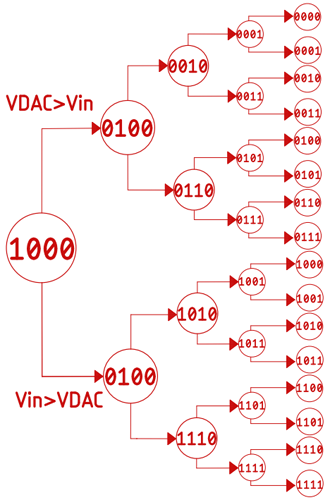### Conversion Time, Speed, and Resolution of Successive Approximation ADC

Conversion Time:

In general, we can say that for an N bit ADC, it will take N clock cycles, which means the conversion time of this ADC will become-

`Tc = N x Tclk`

*Tc is short for Conversion Time.

And unlike other ADCs, the conversion time of this ADC is independent of the input voltage.

As we are using a 4-bit ADC, to avoid aliasing effects, we need to take a sample after 4 consecutive clock pulses.

Conversion Speed:

The typical conversion speed of this type of ADC is around 2 - 5 Mega Samples Per Seconds(MSPS), but there are few which can reach up to 10 (MSPS). An example would be LTC2378 by Linear Technologies.

Resolution:

The resolution of this type of ADC can be around 8 - 16 bits, but some types can go up to 20-bits, an example can be ADS8900B by Analog Devices.